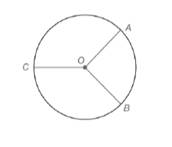Chapter 6.1, Problem 9EElementary Geometry For College St...

7th Edition
Alexander + 2 others
ISBN: 9781337614085

Solutions

Chapter
SectionElementary Geometry For College St...

7th Edition
Alexander + 2 others
ISBN: 9781337614085
Textbook Problem

Given: A O ¯ ⊥ O B ¯  and  O C ¯  bisects  A C B ⌢  in  ⊙ OFind: a) m A B ⌢ b) m A C B ⌢ c) m B C ⌢ d) m ∠ A O C

To determine

(a)

To Find:

If AO¯OB¯ and OC¯ bisects ACB in O find mAB.

Explanation

Given:

The provided figure is given below:

Postulate used:

Central angle postulate:

In a circle, the degree of a central angle is equal to the degree measure of its intercepted arc.

Calculation:

Since, AO¯OB¯ then mAOB=90o.

Therefore, in a circle, the degree of a central angle is equal to the degree measure of its intercepted arc

To determine

(b)

To Find:

If AO¯OB¯ and OC¯ bisects ACB in O find mACB

To determine

(c)

To Find:

If AO¯OB¯ and OC¯ bisects ACB in O find mBC.

To determine

(d)

To Find:

If AO¯OB¯ and OC¯ bisects ACB in O find mAOC.

Still sussing out bartleby?

Check out a sample textbook solution.

See a sample solution

The Solution to Your Study Problems

Bartleby provides explanations to thousands of textbook problems written by our experts, many with advanced degrees!

Get Started

Prove that limx0x2cos(1/x2)=0.

Single Variable Calculus: Early Transcendentals, Volume I

Evaluate the expression sin Exercises 116. (23)2

Finite Mathematics and Applied Calculus (MindTap Course List)

In Exercises 1-10, determine which of the matrices are stochastic. [.4.7.6.3]

Finite Mathematics for the Managerial, Life, and Social Sciences

limx0cscx= a) 0 b) c) d) does not exist

Study Guide for Stewart's Single Variable Calculus: Early Transcendentals, 8th

Describe and explain the independent relationship between main effects and interactions.

Research Methods for the Behavioral Sciences (MindTap Course List)

Define the reliability of measurement and explain why and how it is measured.

Research Methods for the Behavioral Sciences (MindTap Course List)